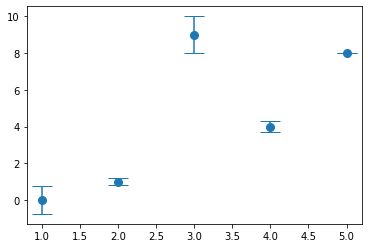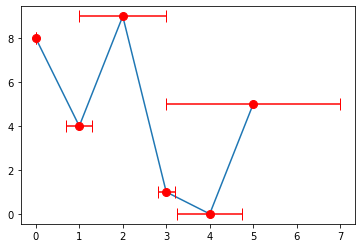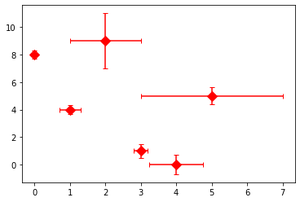# Add perpendicular caps to error bars in Matplotlib

• Last Updated : 26 Dec, 2020

Prerequisites: Matplotlib

The errorbar() function in pyplot module of matplotlib library is used to plot y versus x as lines and/or markers with attached errorbars. For our requirement we need to specifically focussing on capsize attribute of this function. Simply providing a value to it will produce our required functionality.

Attention geek! Strengthen your foundations with the Python Programming Foundation Course and learn the basics.

To begin with, your interview preparations Enhance your Data Structures concepts with the Python DS Course. And to begin with your Machine Learning Journey, join the Machine Learning - Basic Level Course

Syntax: matplotlib.pyplot.errorbar(x, y, yerr=None, xerr=None, fmt=”, ecolor=None, elinewidth=None, capsize=None, barsabove=False, lolims=False, uplims=False, xlolims=False, xuplims=False, errorevery=1, capthick=None, \*, data=None, \*\*kwargs)

Parameters: This method accept the following parameters that are described below:

• x, y: These parameters are the horizontal and vertical coordinates of the data points.
• fmt: This parameter is an optional parameter and it contains the string value.
• capsize: This parameter is also an optional parameter. And it is the length of the error bar caps in points with default value NONE.
• barsabove: This parameter is also an optional parameter. It contains boolean value True for plotting errorsbars above the plot symbols.Its default value is False.
• errorevery: This parameter is also an optional parameter. They contain integer values which is used to draw error bars on a subset of the data.

### Approach

• Import module
• Create data
• Provide error values
• Pass all the values to errorbar() function along with capsize attribute and its value
• Display plot

Example 1:

## Python3

 `import` `matplotlib.pyplot as plt`` ` `x_values ``=` `[``5``, ``4``, ``3``, ``2``, ``1``]``y_values ``=` `[``8``, ``4``, ``9``, ``1``, ``0``]`` ` `y_error ``=` `[``0``, ``0.3``, ``1``, ``0.2``, ``0.75``]`` ` `plt.errorbar(x_values, y_values,  yerr``=``y_error,``             ``fmt``=``'o'``, markersize``=``8``, capsize``=``10``)`` ` `plt.show()`

Output:Example 2:

## Python3

 `import` `matplotlib.pyplot as plt`` ` `x_values ``=` `[``0``, ``1``, ``2``, ``3``, ``4``, ``5``]``y_values ``=` `[``8``, ``4``, ``9``, ``1``, ``0``, ``5``]`` ` `plt.plot(x_values, y_values)``x_error ``=` `[``0``, ``0.3``, ``1``, ``0.2``, ``0.75``, ``2``]`` ` `plt.errorbar(x_values, y_values,  xerr``=``x_error,``             ``fmt``=``'o'``, markersize``=``8``, capsize``=``6``, color``=``"r"``)`` ` `plt.show()`

Output:Example 3:

## Python3

 `import` `matplotlib.pyplot as plt`` ` `x_values ``=` `[``0``, ``1``, ``2``, ``3``, ``4``, ``5``]``y_values ``=` `[``8``, ``4``, ``9``, ``1``, ``0``, ``5``]`` ` ` ` `x_error ``=` `[``0``, ``0.3``, ``1``, ``0.2``, ``0.75``, ``2``]``y_error ``=` `[``0.3``, ``0.3``, ``2``, ``0.5``, ``0.7``, ``0.6``]`` ` `plt.errorbar(x_values, y_values,  xerr``=``x_error, yerr``=``y_error,``             ``fmt``=``'D'``, markersize``=``8``, capsize``=``3``, color``=``"r"``)`` ` `plt.show()`

Output:My Personal Notes arrow_drop_up